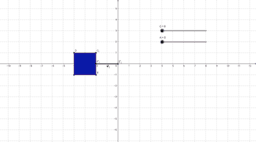Mr Baker

•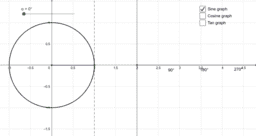Unit circle demo

Activity

Mr Baker

•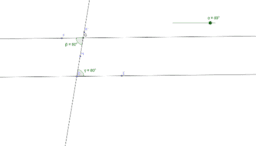Alternate Angle Theorem

Activity

Mr Baker

•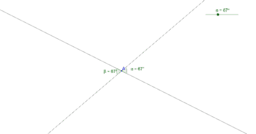Opposite angles at a vertex

Activity

Mr Baker

•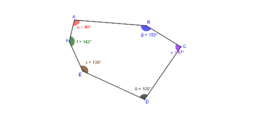Angles in a hexagon

Activity

Mr Baker

•Angles in a pentagon

Activity

Mr Baker

•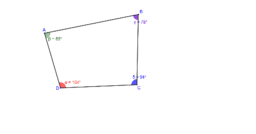Activity

Mr Baker

•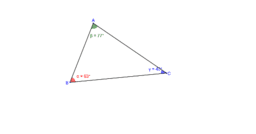Angles in a triangle

Activity

Mr Baker

•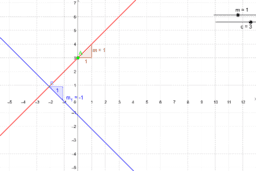Activity

Mr Baker

•Opposite angles at a vertex

Activity

Mr Baker

•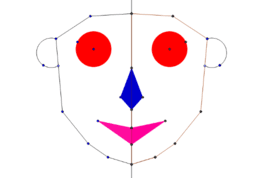Self-portrait

Activity

Mr Baker

•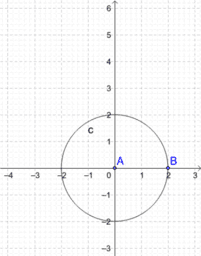Equation of a circle

Activity

Mr Baker

•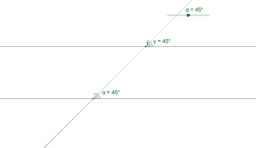Corresponding Angles

Activity

Mr Baker

•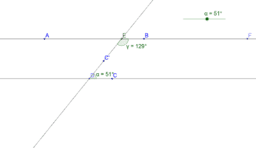Co-interior Angles

Activity

Mr Baker

•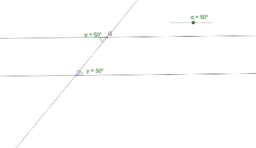Alternate Angles

Activity

Mr Baker

•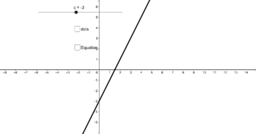y = 2x + c

Activity

Mr Baker

•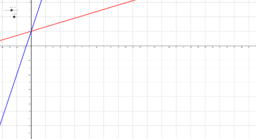y = mx + c

Activity

Mr Baker

•Rotation of square about (0,0) (harder).

Activity

Mr Baker

•